9electrical measuring instruments, electric engines
Оценка 4.7

# 9electrical measuring instruments, electric engines

Оценка 4.7
docx
07.05.2020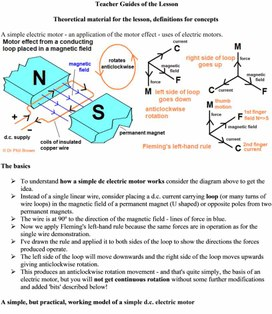9electrical measuring instruments, electric engines.docx

Teacher Guides of the Lesson

Theoretical material for the lesson, definitions for concepts

A simple electric motor - an application of the motor effect - uses of electric motors.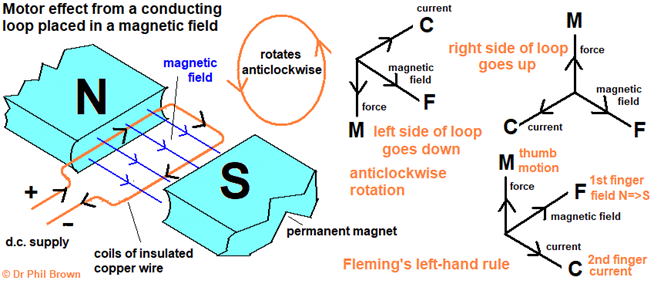The basics

Ø  To understand how a simple dc electric motor works consider the diagram above to get the idea.

Ø  Instead of a single linear wire, consider placing a d.c. current carrying loop (or many turns of wire loops) in the magnetic field of a permanent magnet (U shaped) or opposite poles from two permanent magnets.

Ø  The wire is at 90o to the direction of the magnetic field - lines of force in blue.

Ø  Now we apply Fleming's left-hand rule because the same forces are in operation as for the single wire demonstration.

Ø  I've drawn the rule and applied it to both sides of the loop to show the directions the forces produced operate.

Ø  The left side of the loop will move downwards and the right side of the loop moves upwards giving anticlockwise rotation.

Ø  This produces an anticlockwise rotation movement - and that's quite simply, the basis of an electric motor, but you will not get continuous rotation without some further modifications and added 'bits' described below!

A simple, but practical, working model of a simple d.c. electric motor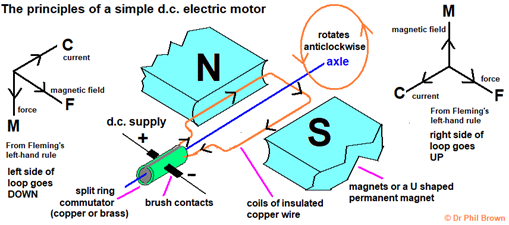Explaining how a simple dc electric motor works

However, as described above, the 'diagram' needs a few more bits to be a working electric motor!

are an axle (spindle) about which the coil can freely rotate between the poles of a permanent magnet, a split ring commutator that swaps the contacts around every half-turn (swapping the +/-polarity) and keeps the rotation in the same direction, it also enables electrical contact to the external circuit, together with the ...

... brush contacts (of graphite block or copper strip) which enable rotation movement to continue but still maintain a complete electrical circuit - the 'brushes' sweep over the surface of the contacts on the axle, and of course a frame structure to hold all the components in place!

The way the forces operate was explained in the previous diagram, but I have repeated the application of Fleming's left-hand rule to show the coil will rotate anticlockwise.

You can reverse the direction of rotation either by either ..

(i) swapping the polarity of the d.c. supply to change the direction of current flow,

and

(ii) swapping the magnetic poles of the permanent magnet to change the direction of the magnetic field.

However, there are several sources of energy loss - decreasing the efficiency of the motor

(a) When the electric motor starts running the current decreases a little from its initial value.

As the current flows, the thin wire coils act as a resistance, the coil heats up a little as heat energy is lost: electrical energy ==> thermal energy store of the motor and surroundings.

Since the temperature of the coils increases, its resistance increases a bit more, leading to a greater increase in wasted energy.

(b) Although this machine is acting as an electric motor, simultaneously it acts as a generator!

As the coil rotates in the magnetic field it induces a current to flow in the opposite direction.

How can you make a simple dc (or any) electric motor more powerful?

There are three ways to do this, all involve increasing the strength of the magnetic field ...

(i)  Increasing the number of turns of wire in the coil.

The magnetic lines of force 'cut' through more wire per unit time.

(ii) By winding the coil on a soft-iron armature to increase the magnetic flux. through the coil.

The ion concentrates the lines of force, so more lines of force are 'cut' through per unit time.

(iii) By making the field magnet as strong as possible.

The stronger the magnet, the greater the magnetic flux - the lines of force are closer together, so

more lines of force are 'cut' per time as the armature rotates.

(iv) Increasing the p.d. across the coil to increase the current.

Increase the charge flow will intensify and strengthen the magnetic field around the coil.

These factors apply to any electric motor design.

These factors can be used to increase the speed of rotation of the motor.

To make an electric motor less powerful or slow its rotation down, (i) reduce the current (by reducing the pd across the coils), (ii) reduce the number of turns of wire coils and (iii) decrease the strength of the magnet to reduce the magnetic flux density.

Factor (i) is used to control the speed of an electric motor e.g. an electric car or train. You can't really change any other factor in a working machine!

Working Principle of Electric Motor

An electric motor is a machine which converts electrical energy into mechanical energy.

Principle:

It is based on the principle that when a current-carrying conductor is placed in a magnetic field, it experiences a mechanical force whose direction is given by Fleming's Left-hand rule and whose magnitude is given by

Force, F = B I l newton

Where B is the magnetic field in weber/m2.

I is the current in amperes and

l is the length of the coil in meter.

The force, current and the magnetic field are all in different directions.

If an Electric current flows through two copper wires that are between the poles of a magnet, an upward force will move one wire up and a downward force will move the other wire down.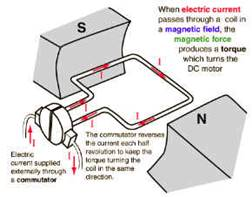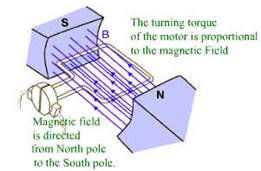Figure 1: Force in DC Motor Figure 2 : Magnetic Field in DC Motor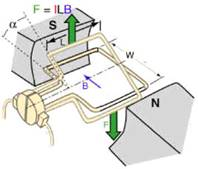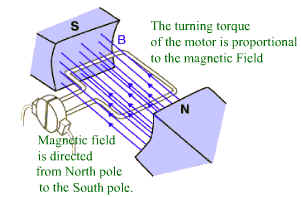Figure 3 :  Torque in DC Motor Figure 4 : Current Flow in DC Motor

The loop can be made to spin by fixing a half circle of copper which is known as commutator, to each end of the loop. Current is passed into and out of the loop by brushes that press onto the strips. The brushes do not go round so the wire do not get twisted.  This arrangement also makes sure that the current always passes down on the right and back on the left so that the rotation continues. This is how a simple Electric motor is made.

Working Principle of the Electrical measuring instruments

The principal of working is same as that of working of a d.c motor. Whenever, a current carrying coil is kept in the magnetic field, it experiences a force and it tends to move. When the current to be measured is made to flow through the coil, a deflecting torque proportional to the flux density and dimensions of the coil is produced which makes the coil to move. as the coil moves, the pointer shows deflection on the scale. The deflection torque is proportional to the coils current and the magnitude of the current flowing through the coil is indicated by a pointer which moves over a graduated scale. When the deflecting torque is equal to the controlling torque, a balance is attained and the pointers shows zero deflection. Here,as the deflection is directly proportional to the current in the coil, uniform scale is obtained.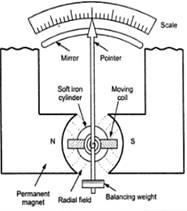Instructions for demonstrations and safety

Warning: experiments with electricity should be performed under the supervision of teachers or adults familiar with electricity safety procedures.

Teacher shows the effect of a magnetic field on a current loop. This is a principle of a simple direct current motor. Show the operational principle of this motor.

·         Wind several turns of wire round a cork and hang it, from a clump, between the poles of magnet as shown. Pass a current briefly through the coil.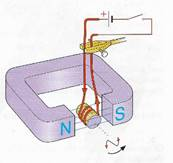ü  What happens?

ü   Does the coil turn the  opposite way if you reverse the current?

There are two ways to find the direction of rotation in the diagram:

Ø  Use Fleming’s Left Hand Rule for each side of the coil.

Find which end of the coil becomes a N-pole. This end will be attracted to the S-pole of the magnet.

Additional guidelines for organizing a lesson

1.      Organization moment. Establishing emotional state.  Checking for absent students.

2.      Teacher introduces the topic and objectives of the lesson, assess criteria.

a.       Teacher demonstrates the force on a current loop using a several turns of wire round a cork

b.      and hang it, from a clump, between the poles of magnet. This is a principle of a simple direct

c.       current motor. Show the operational principle of this motor.

3.      The direction of rotation can be predicted using Fleming’s Left Hand Rule (FLHR).

4.      Teacher sets up apparatus then learners observe to suppose what will happen answer the questions.

5.      Learners are divided into three groups and study deeply the working principle of electricity-measuring instruments, electric motor and a generator at different three station one by one. Teacher put materials at three stations and set a time for each station. Students were given the opportunity to share thinking with each other through group discussions and drawings.

6.      Teacher shows a video clip of the working principle of an electric motor and electric engines.

8.      At the end of the lesson students are encouraged to reflect on what they have learned and

a.       what they need to improve.

Recommendations for formative assessment

Activity1. Students discuss learning objectives and assess criteria.

Activity2. Students with a teacher set up apparatus then observe to suppose what will happen

Activity3. Learners are divided into groups and study deeply the working principle of electricity-

measuring instruments, electric motor and a generator at different three station one by

one. Teacher put materials at three stations and set a time for each station. Students

are given the opportunity to share thinking with each other through group

discussions and drawings. Teacher rotated around to groups to listen, clarify thinking

and prompt further thinking.

As a result of their studying, teacher asks from any students to explain the working

principle of electricity-measuring instruments/electric motor/generator.

Assessment criteria should be agreed in advance.

Activity4. Students individually answer the filling the gap question.

Activity5. At the end of the lesson students are encouraged to reflect on what they have learned

and what they need to improve.

Activity4.

Filling the gap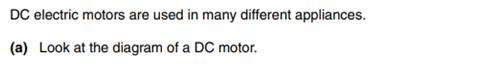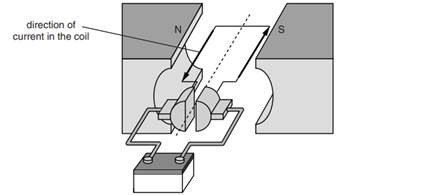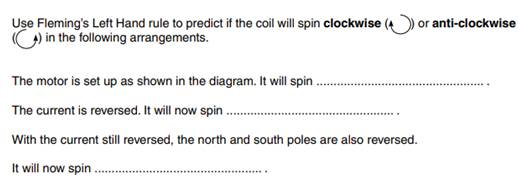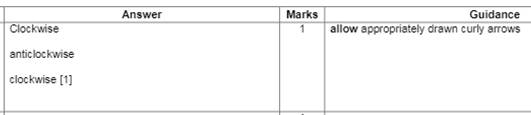List of useful links and literature

Douglas  C. Giancoli, Physics Principles with Applications, Seventh edition  2014.

Keith Johnson, Physics for You, Updated Edition for All GCSE Examinations 2011

Скачано с www.znanio.ru

## The ion concentrates the lines of force, so more lines of force are 'cut' through per unit time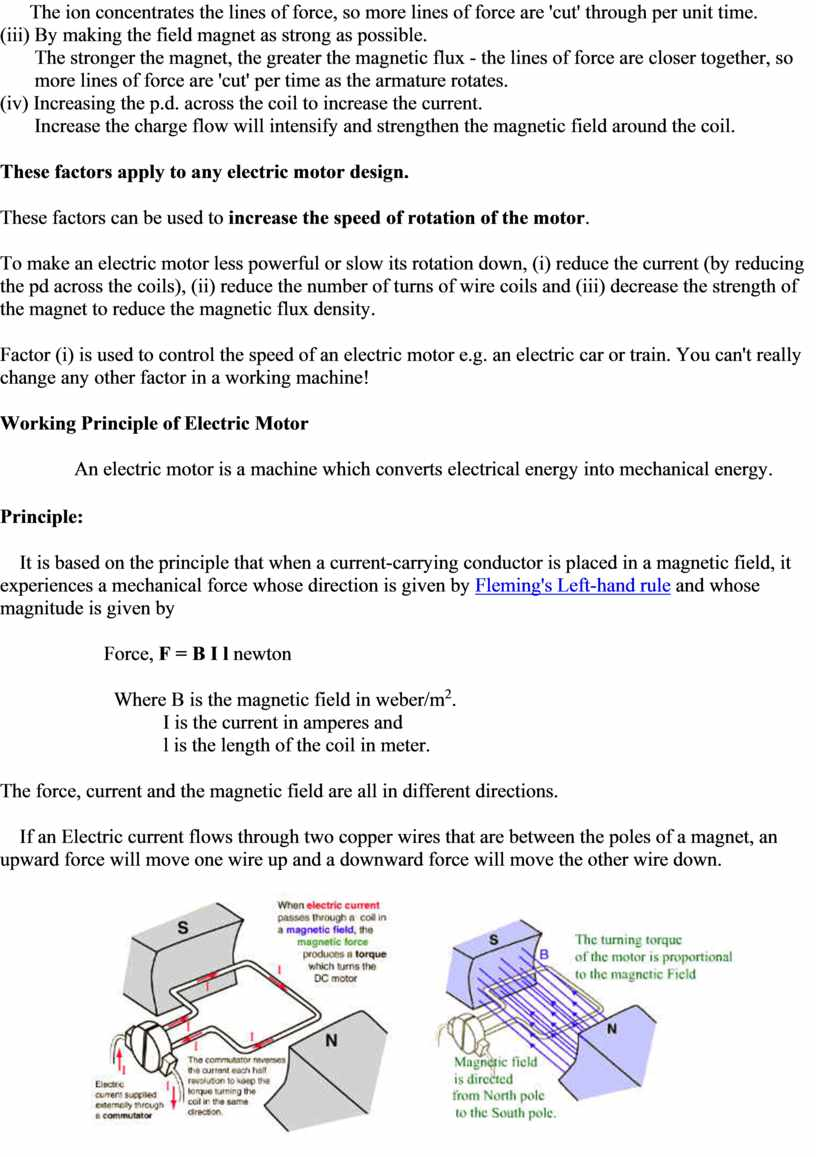## Wind several turns of wire round a cork and hang it, from a clump, between the poles of magnet as shown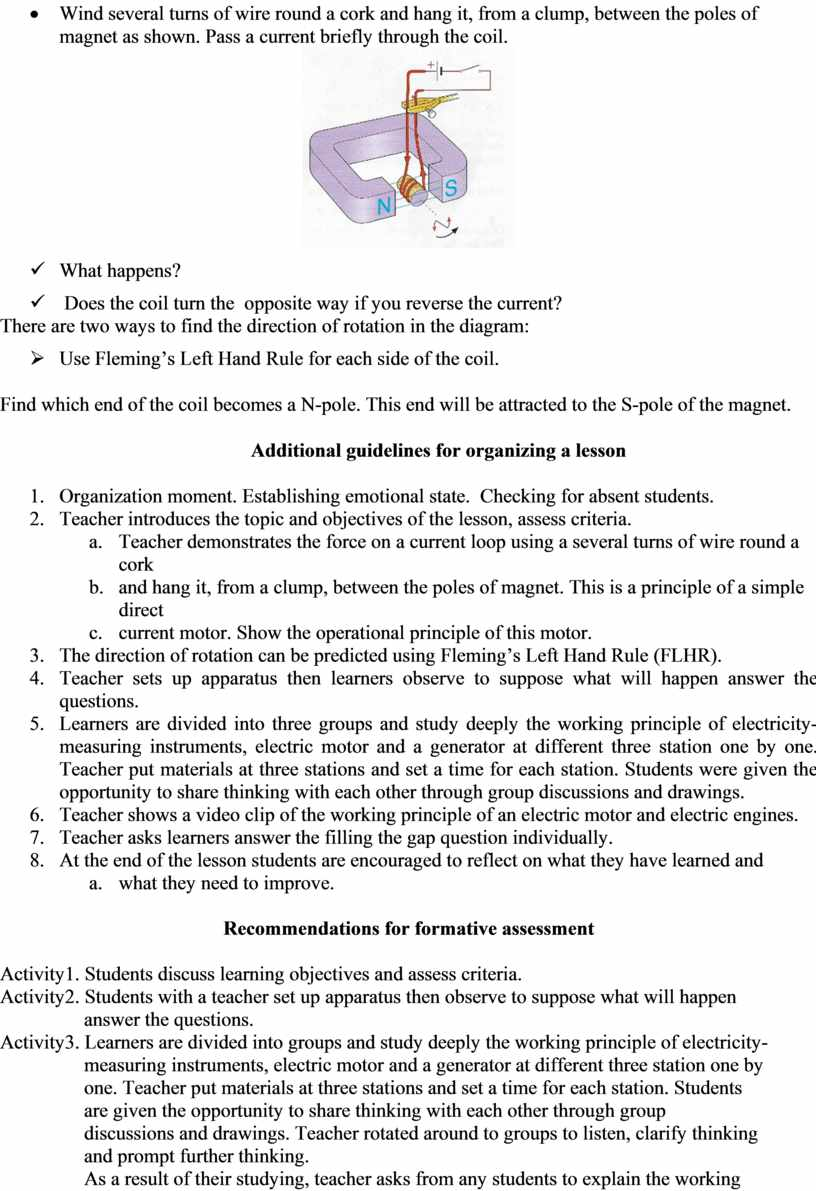Скачать файл